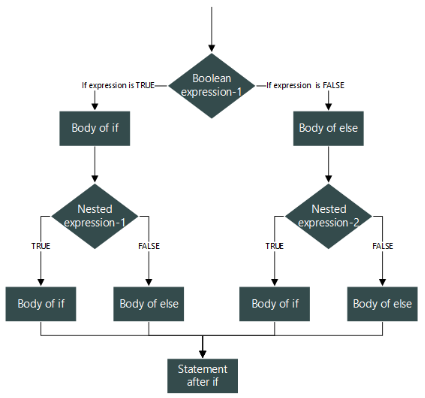#### Nested if else statement

Nested if is a conditional statement, which is used when more than one condition is required to be checked at the same time in a program. The statements are executed from the top to the bottom, checking each condition, whether it meets the criteria or not. If the condition is true, then it executes the block of associated statements of true part, else it goes to the next condition to execute.

Nesting an if means you can use one if or else if statement inside another if or else if statement. The inner if(s) must always be terminated before the outer if(s).#### Example Programs

Program 

Write a program to pass 2 integer numbers as parameters. If either of the two numbers is 0, display invalid entry and the program should end, if it is valid entry, divide the larger number with the smaller number and display the result.

Program 

Write a program to accept three sides of a triangle as parameter and check whether it can form a triangle or not. If it forms a triangle, check whether it is an acute angled, obtuse angled or right-angled triangle.

(Hint: To form a triangle, each side should be less the sum of the other two sides..

To form an acute angled triangle the square of every side should be less than the sum of the squares of the other two sides.

To form an obtuse angled triangle the square of any side should be greater than the sum of the squares of the other two sides.

To form an right angled triangle the square of any side should be equal to the sum of the squares of the other two sides.)

Program 

Write a program to input the three angles of a triangle and check whether it forms a triangle or not, if it forms a triangle, check whether it is an Acute , Obtuse or a Right angled triangle.

(Hint: To form a triangle, the sum of the angles should be 180 degrees.

To form an equilateral triangle every angle should be equal.

To form an isosceles triangle any two angles should be equal.

To form a scalene triangle all three angles should be different from each other.)

Program 

Write a program to input the three sides of a triangle and check whether it forms a triangle or not, if it forms a triangle, check whether it is an equilateral, isosceles or a scalene triangle.

(Hint: To form a triangle, each side should be less the sum of the other two sides.

To form an equilateral triangle every side should be equal.

To form an isosceles triangle any two sides should be equal.

To form a scalene triangle all three sides should be different from each other.)

Program 

Write a program to accept three sides of a triangle as parameter and check whether it can form a triangle or not. If it forms a triangle, check whether it is an acute angled, obtuse angled or right-angled triangle.

(Hint: To form a triangle, each side should be less the sum of the other two sides..

To form an acute angled triangle the square of every side should be less than the sum of the squares of the other two sides.

To form an obtuse angled triangle the square of any side should be greater than the sum of the squares of the other two sides.

To form an right angled triangle the square of any side should be equal to the sum of the squares of the other two sides.)

Program 

A special two-digit number is such that when the sum of its digits is added to the product of

its digits, the result is equal to the original two-digit number.

Example: Consider the number 59.

Sum of digits = 5 + 9 = 14

Product of its digits = 5 x 9 = 45

Sum of the sum of digits and product of digits = 14 + 45 = 59

Write a program to accept a two-digit number. Add the sum of its digits to the product of its

digits.

If the value is equal to the number input, output the message “Special 2-digitnumber” otherwise,

output the message “Not a special2-digit number”.